# Two methods of using pandas to read poorly structured excel. You're welcome to take them away

2022-01-29 16:54:46

Usually , We use Pandas To read Excel data , It is very convenient to convert the data into DataFrame type . But the reality is often very important , When we encounter a structure that is not particularly good Excel When , The conventional Pandas The read operation is not very easy to use , Today, let's look at two reading unconventional structures Excel Examples of data

The test used in this article Excel The contents are as follows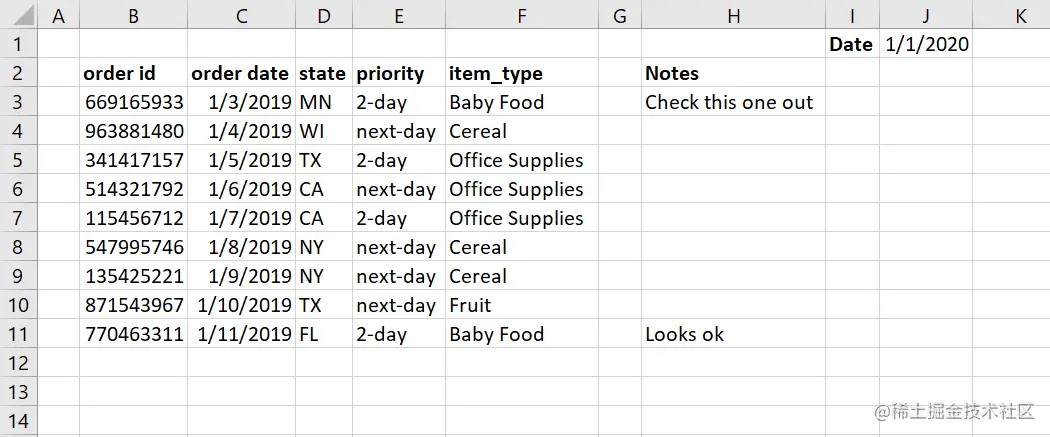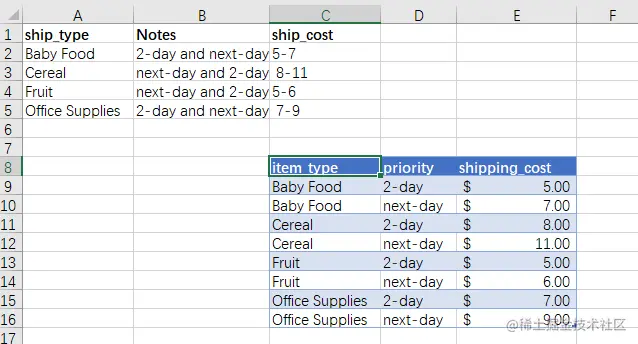The file can be obtained at the end of the text

In general , We use read_excel Function read Excel Data time , All default from the first A Column to start reading , But for some Excel data , Often not from the first A Columns have data , At this point, we need parameters usecols To circumvent

Like the one above Excel data , If we use it directly read_excel(src_file) Read , We will get the following results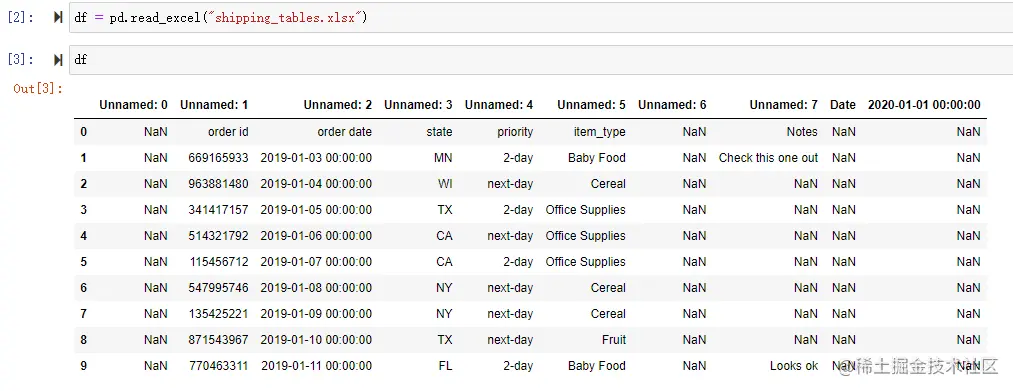We get a lot of unnamed columns and a lot of column data we don't need at all

At this point we can go through usecols To specify which columns of data to read

``````from pathlib import Path
src_file = Path.cwd() /  'shipping_tables.xlsx'

Copy code ``````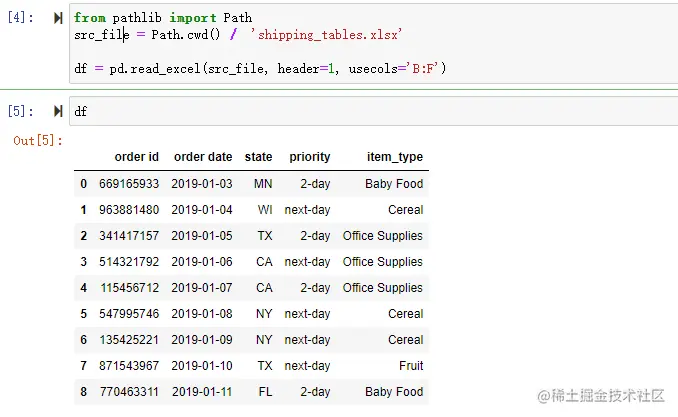You can see the generated DataFrame It only contains the data we need , Deliberately excluded notes Column sum date Field

usecols You can accept a Excel Column scope , for example B:F And read only these columns ,header Parameter requires an integer that defines the title column , Its index is from 0 Start , So we introduced 1, That is to say Excel No 2 That's ok

We can also define a column as a list of numbers

``````df = pd.read_excel(src_file, header=1, usecols=[1,2,3,4,5])
Copy code ``````

You can also select the required column data by column name

``````df = pd.read_excel(
src_file,
usecols=['item_type', 'order id', 'order date', 'state', 'priority'])
Copy code ``````

This is useful when the order of the columns changes but the names of the columns remain the same

Last ,usecols You can also accept a callable function

``````def column_check(x):
if 'unnamed' in x.lower():
return False
if 'priority' in x.lower():
return False
if 'order' in x.lower():
return True
return True

Copy code ``````

This function resolves each column by name , And must return... For each column True or False

You can also use it lambda expression

``````cols_to_use = ['item_type', 'order id', 'order date', 'state', 'priority']
usecols=lambda x: x.lower() in cols_to_use)
Copy code ``````

# Scope and table

In some cases ,Excel The data in may be more uncertain , In our Excel In the data , We have a file named ship_cost Table of , How to get this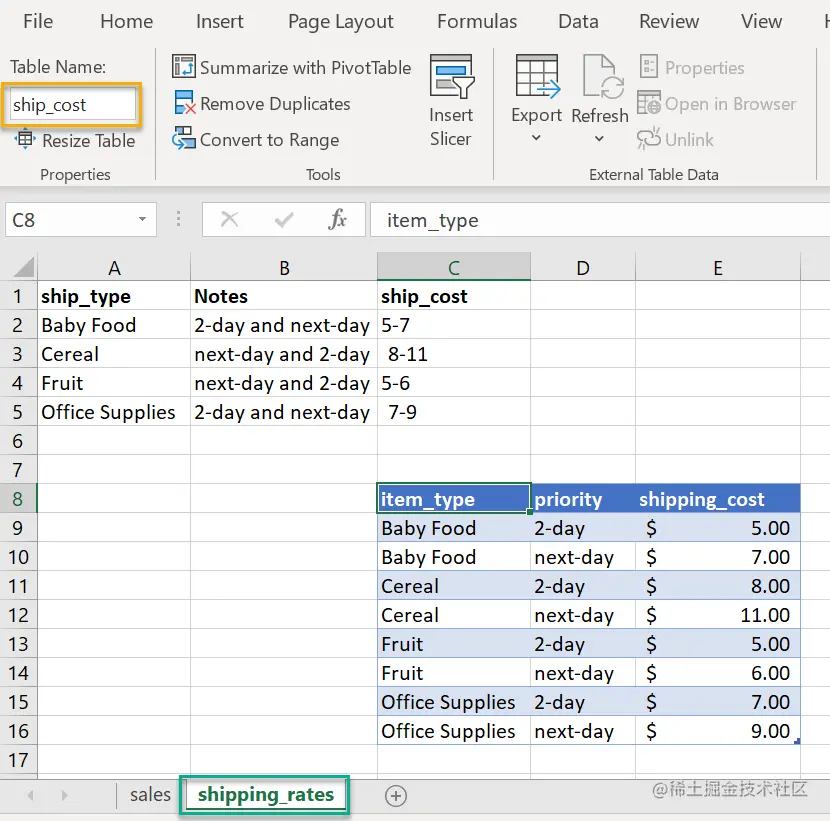under these circumstances , We can use it directly openpyxl Parsing Excel File and convert the data to pandas DataFrame

Here are the USES openpyxl（ After installation ） Read Excel Method of file ：

``````from openpyxl import load_workbook
import pandas as pd
from pathlib import Path
src_file = src_file = Path.cwd() / 'shipping_tables.xlsx'

Copy code ``````

View all sheet page , Get a sheet page , obtain Excel Range data

``````wb.sheetnames
sheet = wb['shipping_rates']
lookup_table = sheet.tables['ship_cost']
lookup_table.ref
Copy code ``````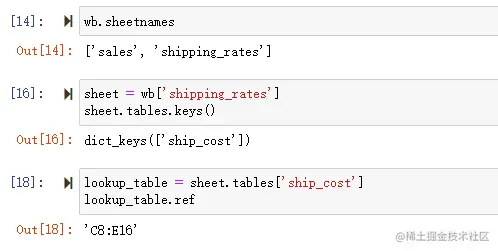Now we know the data range to be loaded , The next step is to convert the range to Pandas DataFrame

``````#  Get data range
data = sheet[lookup_table.ref]
rows_list = []

#  Loop data
for row in data:
cols = []
for col in row:
cols.append(col.value)
rows_list.append(cols)

df = pd.DataFrame(data=rows_list[1:], index=None, columns=rows_list)
Copy code ``````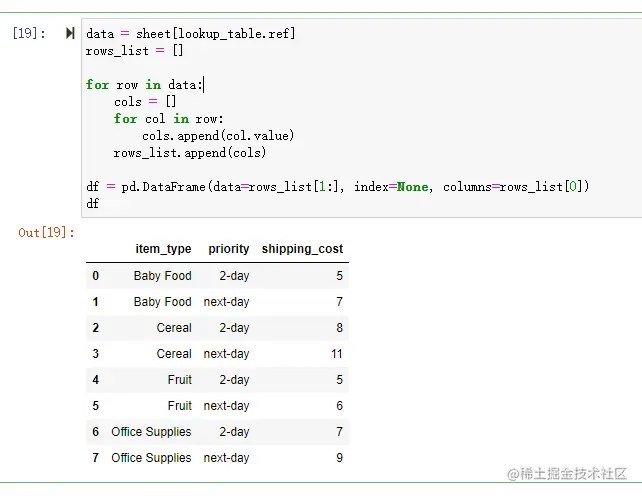In this way, we can get clean table data

Okay , Today's two little knowledge points are shared here , See you next time ！

Need complete code and testing Excel data , Diandian is watching , Wechat access to ！